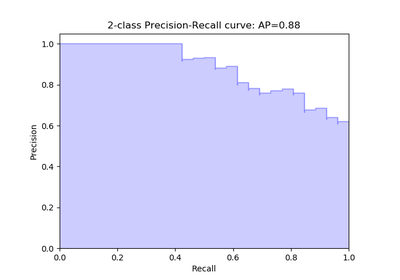# `sklearn.metrics`.precision_recall_curve¶

`sklearn.metrics.``precision_recall_curve`(y_true, probas_pred, pos_label=None, sample_weight=None)[source]

Compute precision-recall pairs for different probability thresholds

Note: this implementation is restricted to the binary classification task.

The precision is the ratio `tp / (tp + fp)` where `tp` is the number of true positives and `fp` the number of false positives. The precision is intuitively the ability of the classifier not to label as positive a sample that is negative.

The recall is the ratio `tp / (tp + fn)` where `tp` is the number of true positives and `fn` the number of false negatives. The recall is intuitively the ability of the classifier to find all the positive samples.

The last precision and recall values are 1. and 0. respectively and do not have a corresponding threshold. This ensures that the graph starts on the x axis.

Read more in the User Guide.

Parameters: y_true : array, shape = [n_samples] True targets of binary classification in range {-1, 1} or {0, 1}. probas_pred : array, shape = [n_samples] Estimated probabilities or decision function. pos_label : int or str, default=None The label of the positive class sample_weight : array-like of shape = [n_samples], optional Sample weights. precision : array, shape = [n_thresholds + 1] Precision values such that element i is the precision of predictions with score >= thresholds[i] and the last element is 1. recall : array, shape = [n_thresholds + 1] Decreasing recall values such that element i is the recall of predictions with score >= thresholds[i] and the last element is 0. thresholds : array, shape = [n_thresholds <= len(np.unique(probas_pred))] Increasing thresholds on the decision function used to compute precision and recall.

`average_precision_score`
Compute average precision from prediction scores
`roc_curve`
Compute Receiver operating characteristic (ROC) curve

Examples

```>>> import numpy as np
>>> from sklearn.metrics import precision_recall_curve
>>> y_true = np.array([0, 0, 1, 1])
>>> y_scores = np.array([0.1, 0.4, 0.35, 0.8])
>>> precision, recall, thresholds = precision_recall_curve(
...     y_true, y_scores)
>>> precision
array([ 0.66...,  0.5       ,  1.        ,  1.        ])
>>> recall
array([ 1. ,  0.5,  0.5,  0. ])
>>> thresholds
array([ 0.35,  0.4 ,  0.8 ])
```

## Examples using `sklearn.metrics.precision_recall_curve`¶Precision-Recall